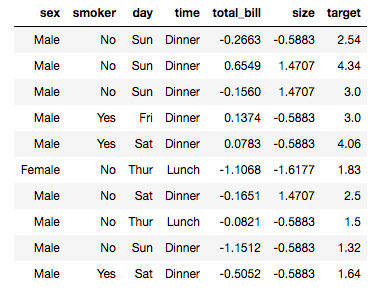# Predicting a Waiter’s Tips

Source: Deep Learning on Medium# Predicting a Waiter’s Tips

Lesson 4 of “Practical Deep Learning for Coders” by fast.ai

In Lesson 4 of “Practical Deep Learning for Coders” by fast.ai, we discover how to how to use deep learning and collaborative filtering to solve tabular data problems. As I always do with the fast.ai lectures, I watched the lecture through once, then watched it again as I ran through the notebooks, pausing as needed. When I finished that, I wanted to make sure I could replicate the process with a different dataset, and I chose the Kaggle dataset “A Waiter’s Tips” by Joe Young. With this dataset, we want to make a model to predict the tip amount for a waiter in a restaurant.

I start by downloading dataset and unzipping it, checking for missing values (there are none!), then uploading the tips.csv file to the ‘data’ folder in the working directory.

After `from fastai.tabular import *` , I need to edit the notebook to make the path point to the right place, since the notebook default path points to the dataset used in the lecture. My data is in the ‘data’ folder, so I set the path accordingly, and tell it to store the data in a Pandas `DataFrame`:

`path = Path(‘data’)df = pd.read_csv(path/’tips.csv’)`

Then I set up the column names, dependent variable, and preprocessing functions. Since I want to predict the tip amount based on the other factors I set `tip` as the dependent variable, and since `sex`, `smoker` status, and`day` of the week can be selected from short lists of possibilities, I set those as “categorical” variables. The `total_bill` and the `size` are just numbers, so those are “continuous” variables. I had to think for a minute about the`time` of day, though: “time” feels like a continuous idea, but looking at the data, I see that “time” is defined as being either “lunch” or “dinner.” Categorical, then.

The preprocessing functions `FillMissing`, `Categorify`, and `Normalize` come with the notebook, and I keep them as they are for now.

`dep_var = 'tip'cat_names = ['sex', 'smoker', 'day', 'time']cont_names = ['total_bill', 'size']procs = [FillMissing, Categorify, Normalize]`

(This California girl was surprised to see ‘smoker’ listed as a possible attribute of a restaurant patron)

Next, I need to choose a size for my test set. We usually set aside 20% of the data for the test set, so since my dataset has 244 elements, I set the test set to use a range of indices from 196–244.

`test = TabularList.from_df(df.iloc[196:244].copy(), path=path, cat_names=cat_names, cont_names=cont_names)`

Then it’s time to use the fastai library’s Datablock API to create my `databunch`:

`data = (TabularList.from_df(df, path=path, cat_names=cat_names, cont_names=cont_names, procs=procs) .split_by_idx(list(range(196,244))) .label_from_df(cols=dep_var) .add_test(test) .databunch())`

I check out a batch of the data:

`data.show_batch(rows=10)`